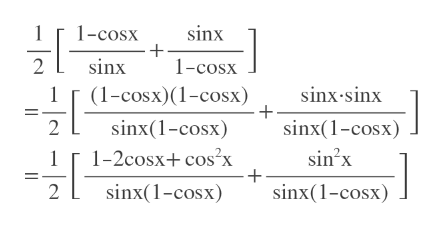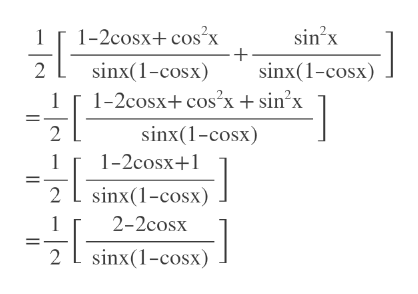# A) How does 1/2[(1-cosx)/(sinx)+(sinx)/(1-cosx)] equal on of these: secx, cosx, sinx, tanx, cotx, cosx, or 1 B) How does ±√((csc^2x-1)/(csc^2x)) equal on of these: secx, cosx, sinx, tanx, cotx, cosx, or 1

Question
3 views

A) How does 1/2[(1-cosx)/(sinx)+(sinx)/(1-cosx)] equal on of these: secx, cosx, sinx, tanx, cotx, cosx, or 1

B) How does ±√((csc^2x-1)/(csc^2x)) equal on of these: secx, cosx, sinx, tanx, cotx, cosx, or 1

check_circle

Step 1

Since you have submitted two questions, we'll answer the first question. For the second question please submit the question again and specify it.

We need LCD.

LCD=sinx*(1-cosx)

Multiply first fraction by (1-cosx) and second by sinx in mumerator and denominator.

Then simplify.help_outlineImage Transcriptionclose1-cosx sinx 2 sinx 1-cosx (1-cosx) (1-cosx) sinx-sinx sinx(1-cosx) 2 sinx(1-cosx) sin2x 1-2cosx+ cos'x 1 sinx(1-cosx) 2 sinx(1-cosx) fullscreen
Step 2

Add the numerators [since denominators are same]

Ap...help_outlineImage Transcriptionclose1-2cosx+ cos'x + sinx(1-cosx) sinx 2 2 sinx(1-cosx) 1-2cosx cos2x + sin?x 2 sinx(1-cosx) 1-2cosx+1 2 sinx(1-cosx) 2-2cosx 2 sinx(1-cosx) fullscreen

### Want to see the full answer?

See Solution

#### Want to see this answer and more?

Solutions are written by subject experts who are available 24/7. Questions are typically answered within 1 hour.*

See Solution
*Response times may vary by subject and question.
Tagged in

### Other